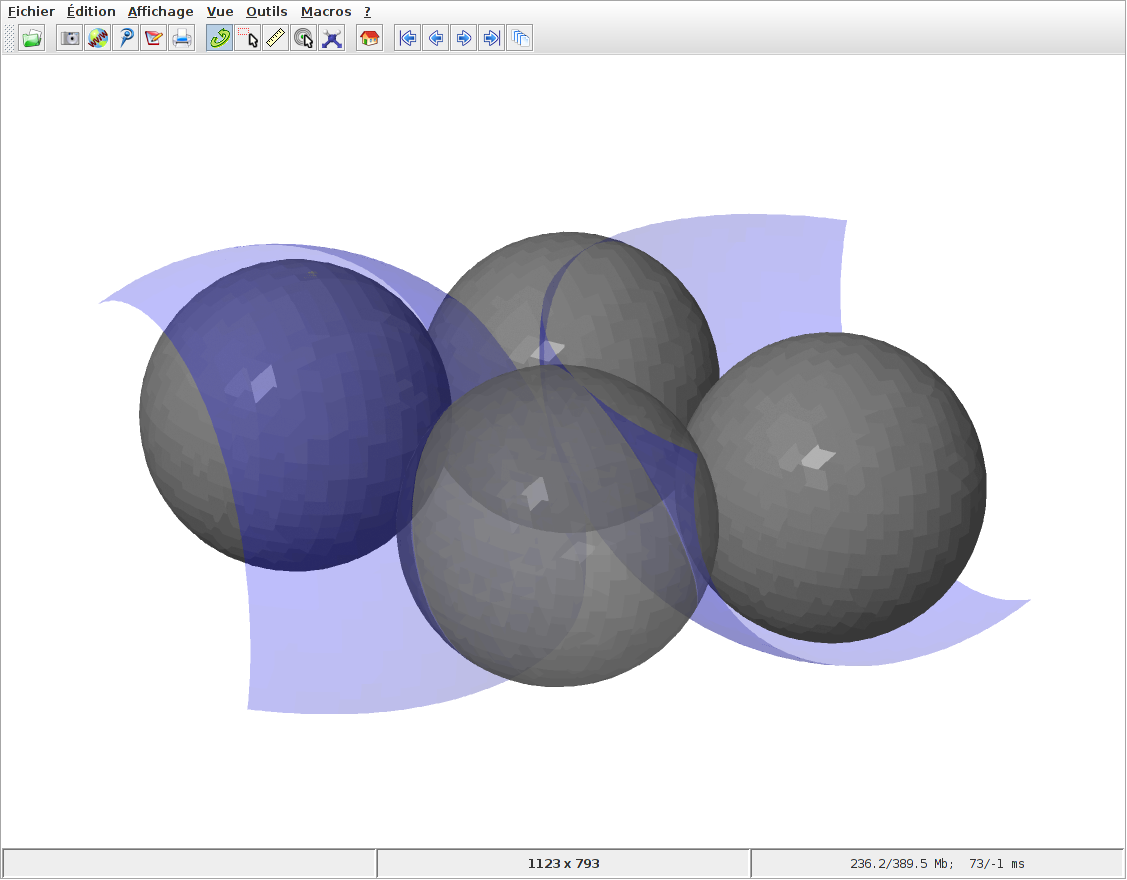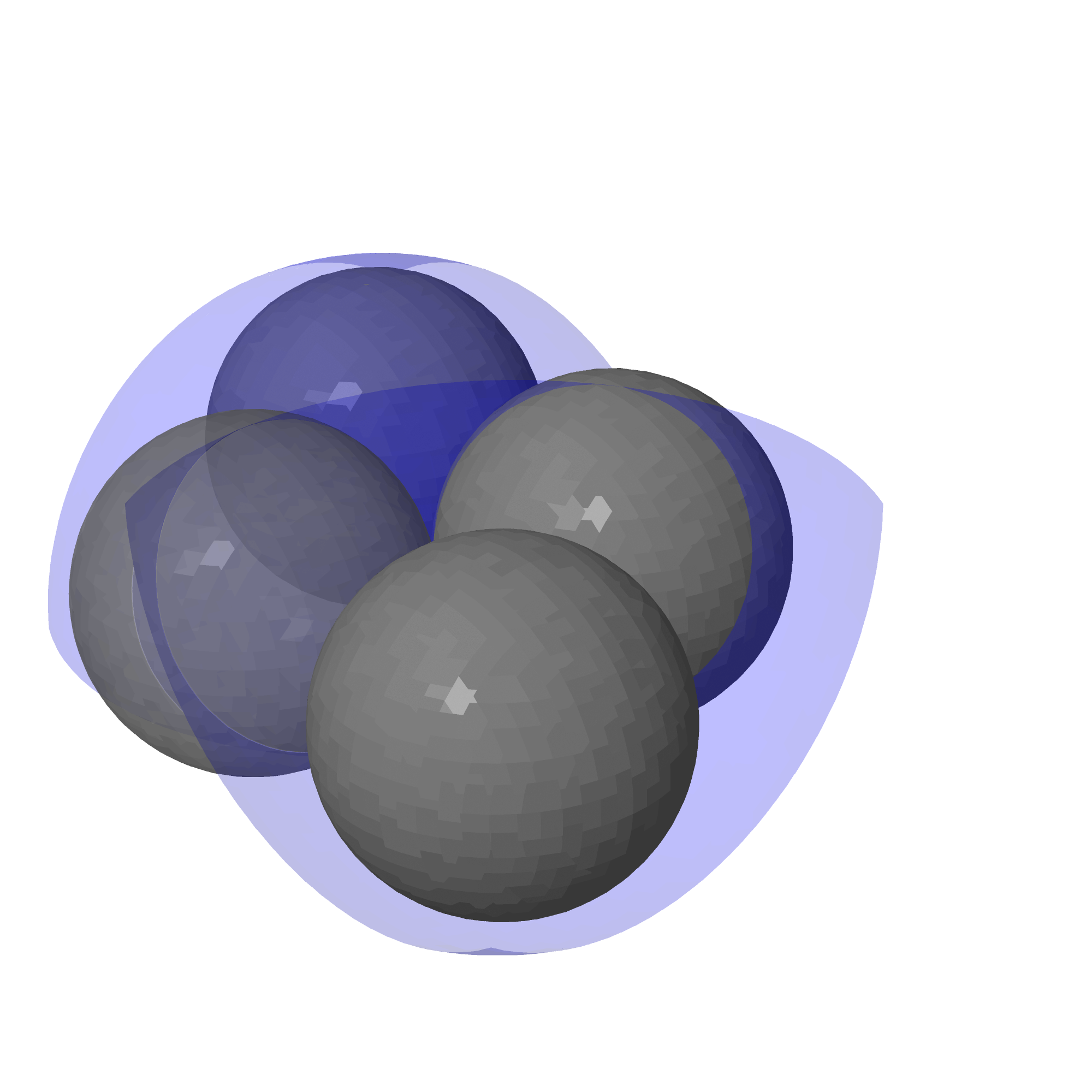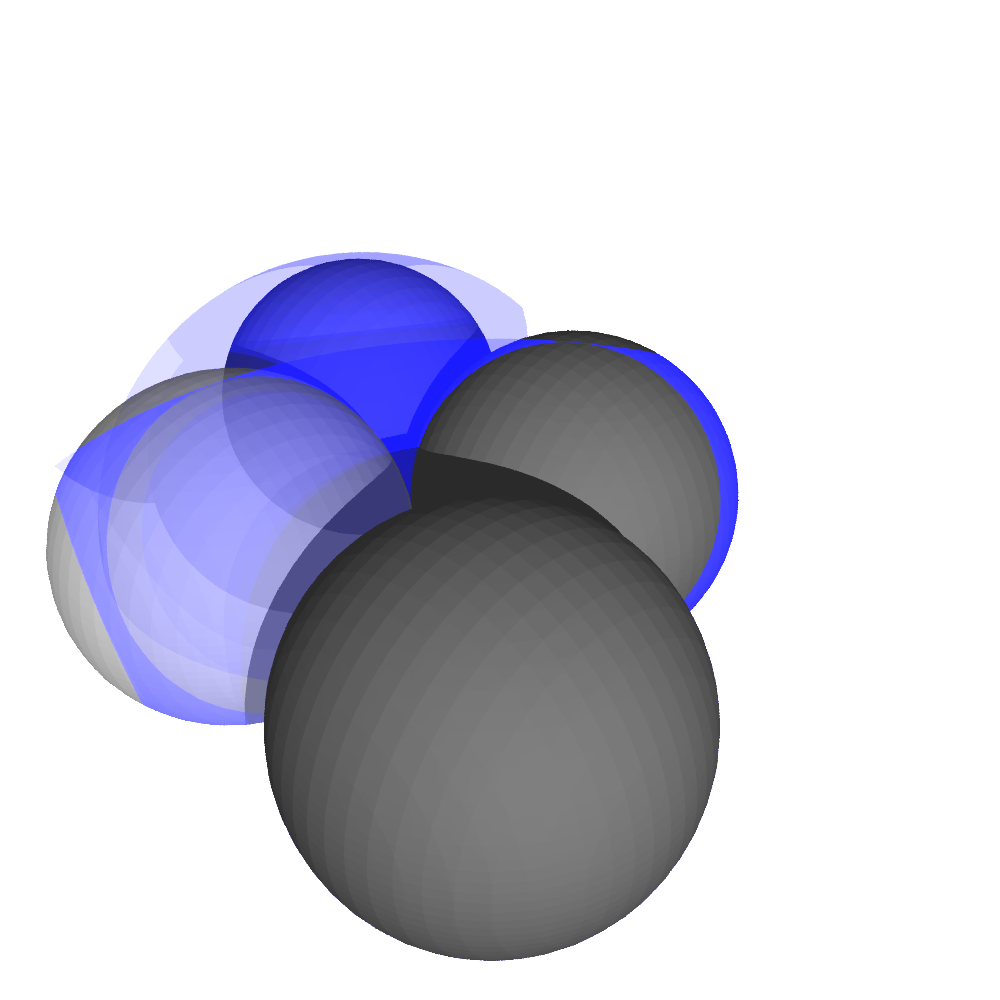3d plot, slow

I have some graphical objects (spheres and planes) and I'm trying to create a nice picture to export as a .png (other options?). The problem I'm having is that the interactive mode (right clicking on the graphic) is so slow that the menu options freeze up. I'd like to preview the image (rotate it around, zoom, etc.) before saving so that I can make sure I've got the right picture. What is a more efficient/ faster way to create and export a 3d picture? Thanks!

var('x,y,z')

E1 = implicit_plot3d(x^2+y^2+z^2==1/4, (x,-1/2,3/2), (y,-1/2,sqrt(3)/2+1/2), (z,-0.5,0.5), color = 'gray', opacity = 0.6)

E2 = implicit_plot3d((x-1)^2+y^2+z^2==1/4, (x,-1/2,3/2), (y,-1/2,sqrt(3)/2+1/2), (z,-0.5,0.5), color = 'gray')

E3 = implicit_plot3d((x-1/2)^2+(y-sqrt(3)/2)^2+z^2==1/4, (x,-1/2,3/2), (y,-1/2,sqrt(3)/2+1/2), (z,-0.5,0.5), color = 'gray')

E4 = implicit_plot3d(z == -1/2, (x,-1,4), (y,-1,4), (z,-0.6,0.5), color = 'gray', opacity = 0.2)

E6 = implicit_plot3d((x+1/2)^2+(y-sqrt(3)/2)^2+z^2==1/4, (x,-1,3/2), (y,-1/2,sqrt(3)/2+1/2), (z,-0.5,0.5), color = 'gray')

S1 = implicit_plot3d((x-1)^2+y^2+(z-1/2)^2==1, (x,-1,3/2), (y,-1/2,sqrt(3)/2+1/2), (z,-0.5,0.5), color = 'blue', opacity = 0.2)

S2 = implicit_plot3d((x+1/2)^2+(y-sqrt(3)/2)^2+(z+1/2)^2==1, (x,-1,3/2), (y,-1/2,sqrt(3)/2+1/2), (z,-0.5,0.5), color = 'blue', opacity = 0.2)

show(E1+E2+E3+E6+M1+S1+S2, aspect_ratio = 1, frame = false)

Also, if I try

t = E1+E2+E3+E6+M1+S1+S2

t.save('spheres.png', aspect_ratio = 1, frame = false) then the picture is quite distorted. Is aspect_ratio not what I want for 3d plots?

edit retag close merge delete

Also, is there a way to crank up the resolution so the spheres don't look pixelated?

Sort by » oldest newest most voted

First, you may look at the documentation which list the kind of arguments you can try:

sage: S = sphere()
sage: S.show?
sage: S.save?

The variable M1 is not defined in the question so show(E1+E2+E3+E6+M1+S1+S2, aspect_ratio = 1, frame = false) returns NameError: name 'M1' is not defined on my computer. Removing M1 from the sum, I am able to run show in about 1s (running on a recent computer with Ubuntu 16.04):

sage: %time show(E1+E2+E3+E6+S1+S2, aspect_ratio=1, frame=False)
Launched jmol viewer for Graphics3d Object
CPU times: user 172 ms, sys: 16 ms, total: 188 ms
Wall time: 1.01 sThen, using zoom and figsize arguments I get for Jmol viewer:

sage: t = E1+E2+E3+E6+S1+S2 # +M1
sage: %time t.save('spheres-jmol.png', aspect_ratio=1, frame=False, zoom=1.5, figsize=20, viewer='jmol')
CPU times: user 164 ms, sys: 8 ms, total: 172 ms
Wall time: 1.36 sand for Tachyon viewer:

sage: %time t.save('spheres-tachyon.png', aspect_ratio=1, frame=False, zoom=1.4, figsize=10, viewer='tachyon')
CPU times: user 64 ms, sys: 8 ms, total: 72 ms
Wall time: 2.23 sYou may want to test the other viewers (see the documentation I have mentionned above).

more